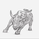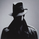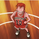TradingView35631 obejrzeń
As we all know, as published by Wilder, RSI makes use of "CLOSE" values. You probably have experimented changing the input to hl2 or hlc3 . I have included many other RSI variations in this chart. Refer to the developers section below to learn how you can use this code in your scripts.

1) RSI with Volume
---------------------------------------------
Suggested by Morris, this idea adds volume to the RSI indicator. Because volume offers one means of determining whether money is entering or leaving a market, this would provide additional information with which to make trading decisions.

2) RSI using last Open
---------------------------------------------
This is RSI with yesterday's open, This basically compares two full days of price action and in the process produces a smoother RSI line.

RSI of today's close is used as a signal (blue line).

3) RSI using SMA
---------------------------------------------
Wilder used his own MA for calculating RSI (check my post on Wilders MA here - -- This closely resembles EMA ). One of Morris's suggestion is to try out SMA .

Compared to normal RSI , you will see more squiggles here.

4) RSI using EMA
---------------------------------------------
Same idea as above, but using EMA .

5) RSI with Fibs
---------------------------------------------
How much does RSI retrace? This makes it easy to determine that :)

6) RSI of MACD
---------------------------------------------
As I mentioned earlier, RSI is a pluggable formula. You can substitute "close" with any data series to derive an index out of it.

This shows RSI of MACD . Note that this is range bound.

More info on RSI variations:
https://drive.google.com/file/d/0Bx48Du_...

For Pinescript developers:
---------------------------------------------
You can substitute your favorite indicator in the RSI function. I have made the RSI calculation a separate function in all the indicators above.

Following are the reusable functions (simply copy to your script and call with proper arguments):
* WiMA( src , length)
* calc_rsi( fv , length): This is equivalent to stock rsi () in TV.
* calc_rsi_volume( fv , length)
* calc_rsi_sma( fv , length)
* calc_rsi_ema( fv , length)
* calc_rsi_lastopen( fv , length)
* calc_macd( src , fast, slow)

You can also pick up fibs drawing code and put in on any indicator.

Skrypt open-source

Zgodnie z prawdziwym duchem TradingView, autor tego skryptu opublikował go jako open-source, aby traderzy mogli go zrozumieć i zweryfikować. Brawo dla autora! Możesz używać go za darmo, ale ponowne wykorzystanie tego kodu w publikacji jest regulowane przez Dobre Praktyki. Możesz go dodać do ulubionych, żeby używać go na wykresie.

Chcesz użyć tego skryptu na wykresie?
//
// @author LazyBear
//
// If you use this code in its original/modified form, do drop me a note.
//
study("RSI + Volume [LazyBear]", shorttitle="RSIVolume_LB")
length=input(14)
ob=input(80, title="Overbought")
os=input(20, title="Oversold")

WiMA(src, length) =>
MA_s=(src + nz(MA_s * (length-1)))/length
MA_s

calc_rsi_volume(fv, length) =>
up=iff(fv>fv,abs(fv-fv)*volume,0)
dn=iff(fv<fv,abs(fv-fv)*volume,0)
upt=WiMA(up,length)
dnt=WiMA(dn,length)
100*(upt/(upt+dnt))

rsi_v = calc_rsi_volume(close, length)

u=plot(ob)
l=plot(os)
fill(u,l,red)
plot(50)
plot(rsi_v, color=red, linewidth=1)
List of my free indicators: http://bit.ly/1LQaPK8
List of my indicators at Appstore: http://blog.tradingview.com/?p=970

## KomentarzeRSI of not MACD but MACD signal line crossovers may also be used. I changed the RSI length to 2, used MACD(12,26,9) and then take the inverse fisher transform of the result with the smoothing length 2. Here's the Pinecode:

study("RSI MACD ", shorttitle="RSIMACD_LB")
length=input(2, title="RSI Length")
fast=input(12, title="MACD fast length")
slow=input(26, title="MACD slow length")

WiMA(src, length) =>
MA_s=(src + nz(MA_s * (length-1)))/length
MA_s

calc_rsi(fv, length) =>
up=iff(fv>fv,abs(fv-fv),0)
dn=iff(fv<fv,abs(fv-fv),0)
upt=WiMA(up,length)
dnt=WiMA(dn,length)
100*(upt/(upt+dnt))

calc_macd(fv, fast, slow) =>
fastMA = alma(fv, fast, 0.85, 6)
slowMA = alma(fv, slow, 0.85, 6)
macd = 100*(fastMA - slowMA)/slowMA
signal = alma(macd,9,0.85,6)
macd - signal

lengthwma=input(2, title="Smoothing length")
calc_ifish(series, lengthwma) =>
v1=0.1*(series-50)
v2=wma(v1,lengthwma)
ifish=(exp(2*v2)-1)/(exp(2*v2)+1)
ifish

plot(calc_ifish(calc_rsi(calc_macd(close, fast, slow), length), lengthwma), color=red)
hline(0.5)
hline(-0.5)
OdpowiedzNajska
If anyone tries to implement the script nowadays, it wouldn't work. For some reason, box brackets and their contents are not displayed in comments, so subscript (1) (replace with box brackets) is missing in a few places. WiMA and calc_rsi functions can be easily fixed when you compare it with the LazyBear's code. Everything else works well, thanks for the script!
OdpowiedzHi fellows, would someone share the RSIFibs_LB pine script because I can't find it? Thank you!
OdpowiedzHello, help me please where can I get this RSI indicator with Fibs I don't know how to do that ???!
Odpowiedzarsonist
@arsonist, could you get it ???? Also I couldn't find it :(
Odpowiedz